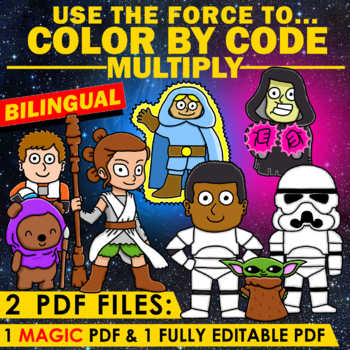# USE THE FORCE to Color by Code – BILINGUAL – Multiply – EDITABLE MAGIC PDF!Subject
Resource Type
Format
Zip (97 MB|48 pages)
Standards
\$6.50
\$6.50

#### Also included in

1. Save money getting all my Star Wars bilingual resources in one single BUNDLE! :)
\$18.00
\$25.00
Save \$7.00

### Description

Do your students like Star Wars? With these cool puzzles, your students will enjoy Math at the same time as they review the following Common Core Standards: 3.OA.1 / 3.OA.5 / 3.OA.7 / 4.OA.1 / 4.NBT.5

This set includes 6 multiplication math puzzles. The set also includes 6 answer keys.

***I include the Spanish version and the English version of each puzzle, so you can choose the one that fits your needs :)

***All the pages are EDITABLE so you can also give your students exactly the words they need! Make sure you open these pdf files with Adobe Reader and simply type the words you choose!

To suit everybody´s needs I include 2 PDF files:

PDF file n.1: These are the puzzles in black and white. I also include a full-color version of each puzzle.
This is also a FULLY EDITABLE PDF file. You can customize each puzzle with your text: your students can color by number, color by sight word, color by… YOU DECIDE!

PDF file n.2: These are the Answer Keys.
This is also an EDITABLE MAGIC PDF file: You just need to enter the words you need 1 time and the coloring picture will be automatically populated with those words. It´s so easy :)

After the children have colored the puzzles, you can cut out the picture portion of each printable to decorate your classroom :)

~~~~~~~~~~~~~~~~~~~~~~~~~~~~~~~~~~~~~~~~~~~~~~~~~~~~~~~~~~~~~~~~~~~~~~~~~~~~~~~~~~~~~~~~~~~~~~~~

CLICK HERE if you only need the ENGLISH VERSION of this set!

*You can check my Pinterest Boards for more cool materials!
MATH
MY TPT STORE

~~~~~~~~~~~~~~~~~~~~~~~~~~~~~~~~~~~~~~~~~~~~~~~~~~~~~~~~~~~~~~~~~~~~~~~~~~~~~~~~~~~~~~~~~~~~~~~~

This product is the work of "Spanish Teacher". It is intended to support the implementation of the CCSS. No approval by, nor association with, the creators of the CCSS is intended or implied.

"The Common Core Standards were written and developed by The National Governors Association Center for Best Practices and Council of Chief State School Officers. Â © Copyright 2010. National Governors Association Center for Best Practices and Council of Chief State School Officers. All rights reserved."

Total Pages
48 pages
Included
Teaching Duration
2 Weeks
Report this Resource to TpT
Reported resources will be reviewed by our team. Report this resource to let us know if this resource violates TpT’s content guidelines.

### Standards

to see state-specific standards (only available in the US).
Interpret a multiplication equation as a comparison, e.g., interpret 35 = 5 × 7 as a statement that 35 is 5 times as many as 7 and 7 times as many as 5. Represent verbal statements of multiplicative comparisons as multiplication equations.
Fluently multiply and divide within 100, using strategies such as the relationship between multiplication and division (e.g., knowing that 8 × 5 = 40, one knows 40 ÷ 5 = 8) or properties of operations. By the end of Grade 3, know from memory all products of two one-digit numbers.
Apply properties of operations as strategies to multiply and divide. Examples: If 6 × 4 = 24 is known, then 4 × 6 = 24 is also known. (Commutative property of multiplication.) 3 × 5 × 2 can be found by 3 × 5 = 15, then 15 × 2 = 30, or by 5 × 2 = 10, then 3 × 10 = 30. (Associative property of multiplication.) Knowing that 8 × 5 = 40 and 8 × 2 = 16, one can find 8 × 7 as 8 × (5 + 2) = (8 × 5) + (8 × 2) = 40 + 16 = 56. (Distributive property.)
Interpret products of whole numbers, e.g., interpret 5 × 7 as the total number of objects in 5 groups of 7 objects each. For example, describe a context in which a total number of objects can be expressed as 5 × 7.
Multiply a whole number of up to four digits by a one-digit whole number, and multiply two two-digit numbers, using strategies based on place value and the properties of operations. Illustrate and explain the calculation by using equations, rectangular arrays, and/or area models.